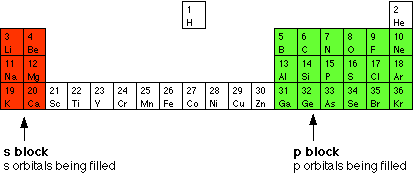# How many p-orbitals are occupied in a K atom?

Jan 28, 2015

Potassium ($\text{K}$) is located in group 1, period 4 of the periodic table and has an atomic number of 19.

Since you're dealing with a neutral atom, the number of electrons $\text{K}$ has must equal 19. You could determine how many p-orbitals are occupied in a $\text{K}$ atom by writing its electron configuration

$\text{K} : 1 {s}^{2} 2 {s}^{2} 2 {p}^{6} 3 {s}^{2} 3 {p}^{6} 4 {s}^{1}$

As you can see, the 2p and 3p sublevels each hold six electrons, which means that they are completely occupied. Since every p sublevel has a total of three p-orbitals - ${p}_{x}$, ${p}_{y}$, and ${p}_{z}$ - the number of p-orbitals occupied in a $\text{K}$ atom is equal to 6 - 3 p-orbitals on the 2p sublevel and 3 p-orbitals on the 3p sublevel.

A faster way to figuring out how many p-orbitals are occupied in a $\text{K}$ atom is by looking at the periodic table. The periodic table is split into blocks, as you can see hereSo, all you have to do is read the periodic table from left to right across each period - much like you would a sentence - until you get to potassium.

If you do this, you'll notice that you've passed two p sublevels: the one that starts at $\text{B}$ and ends at $\text{Ne}$ - the 2p sublevel - and the one that starts at $\text{Al}$ and ends at $\text{Ar}$ - the 3p sublevel. Once again, the conclusion is that $\text{K}$ has 6 p-orbitals which hold 12 of its 19 electrons.